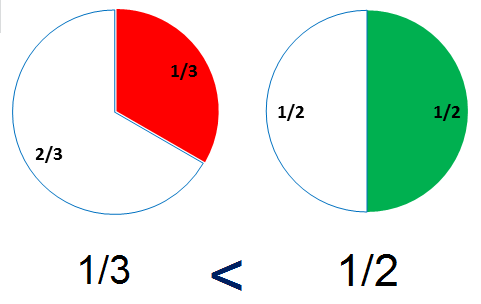# Compare

Identify fractions on number linesComparing Fractions using Models

Example:

Compare 1/3 and  1/2  using circle models.Compare 1/3 and  1/2  using the number lineFractions get larger from left to right.  (least –> greatest)

Comparing fractions using their decimal equivalents.

To convert fractions to decimals you just divide the numerator by the denominator.### Cross Products of Equal Fractions### Cross multiply to compare fractions.

Rule:Example:These fractions are equivalent.Comparing Fractions Help